# Utilize OT/Done Columns in Clocking Schedules

Introduction
You will find the attendance data of users especially work time, over time, short etc will change if you do different configurations in clocking schedule. This might be due to the OT/ Done columns in the clocking schedule.

Benefit
This feature allow you to set whether to calculate overtime automatically without setting a time into schedule OR calculate overtime by fixed time assign in clocking schedule.

Procedure
Example 1
Clocking schedule without OT/Done Settings in clocking schedule: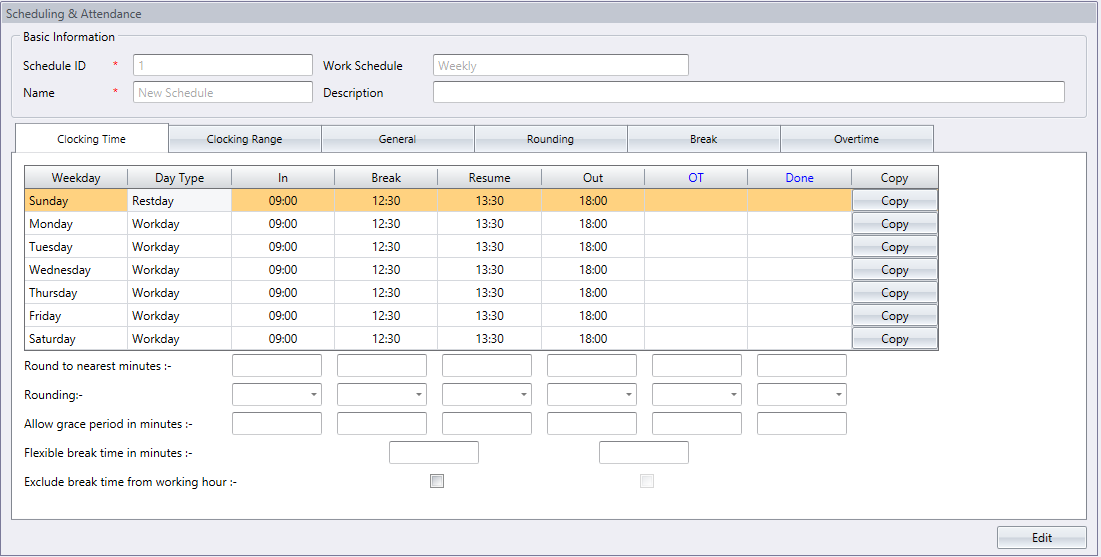Clocking time of user
 Number of clocking 1st 2nd 3rd 4th 5th 6th Cloking time 09:00 12:39 13:15 16:15 19:05 23:40

Result in Attendance Sheet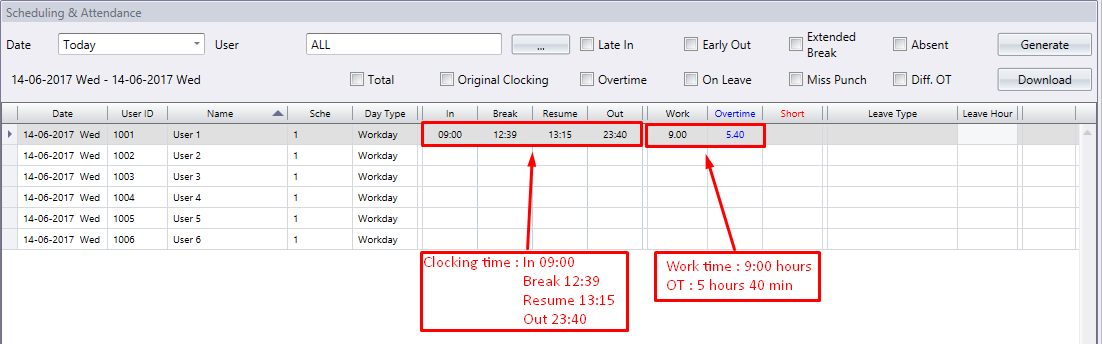There is no preset time available in the OT/ Done column in this clocking schedule. When employee clocks out after the preset out time, 6:00pm, Ingress/TCMS V3 will calculate and show result in his OT column. The logic and calculations of Ingress/TCMS V3 are based on the clock out time of the employee.

If Actual Out time of user > Preset out time
--> OT = Actual Out time – Preset Out time

Actual Out time of user = 11:40pm or 23:40
Preset Out time of user = 6:00pm or 18:00
Therefore  OT = 23:40 – 18:00
= 5 hours 40 min

Ingress/TCMS V3 will do calculation on total working time. Please take note that work time does not include OT of employees.

If Actual Out time of user > Preset Out time
--> Work time = Preset out time – actual in time
Where work time = 18:00 – 09:00
= 9 hours
If Actual Out time of user < Preset Out time
--> Work time = Actual Out time – Actual In time

Example 2
Clocking Schedule with OT/ Done Setting in clocking schedule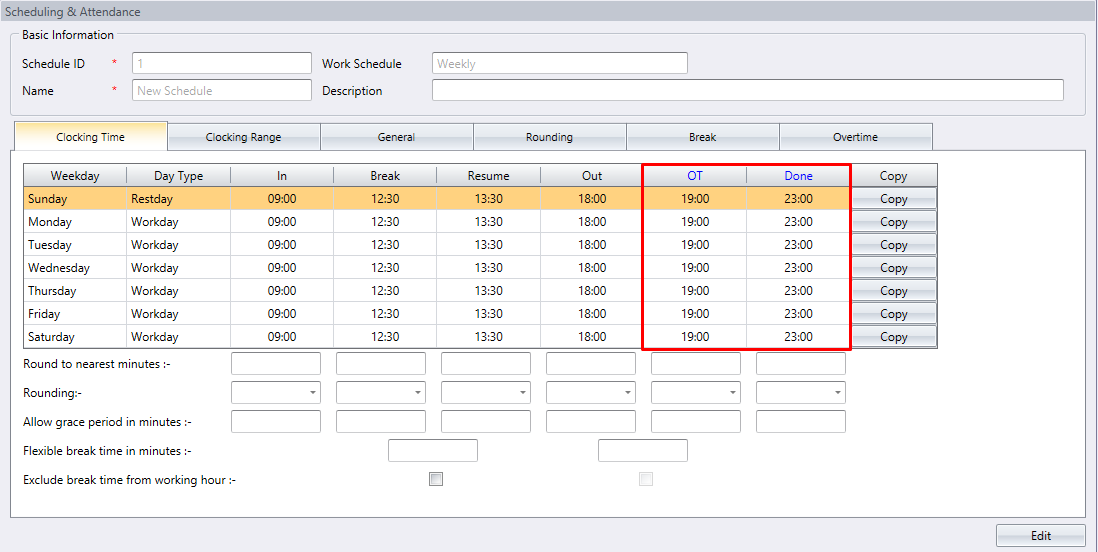Clocking time of user

 Number of clocking 1st 2nd 3rd 4th 5th 6th Cloking time 09:00 12:39 13:15 16:15 19:05 23:40

Result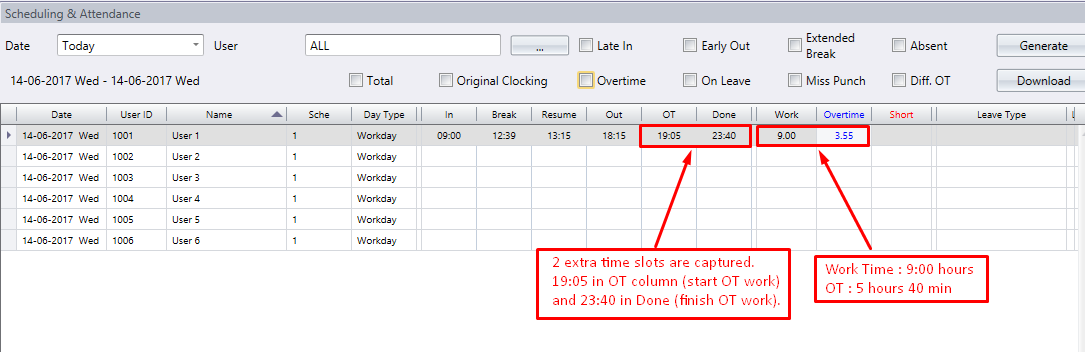Ingress & TCMS V3 will capture the 2 extra clocking times if user configured OT and DONE times in the clocking schedule.
OT is defined as 19:00 in clocking schedule. This means that employee can perform OT work, but the OT will only start at 7:00pm. DONE is defined as 23:00 in clocking schedule. This allows employee to work and claim for OT. Anyway, working time after DONE, 23:00 will not be considered as OT.

 OT DONE Difference Preset 19:00 23:00 4 hours Actual 19:05 23:40 4 hours 35 min Calculated by Ingress/TCMS 19:05 23:40 3 hours 55 min

As preset in clocking schedule, the difference between 19:00 and 23:00 is  4 hours.
The formula is
OT = Preset Done time – Preset OT time
OT = 23:00 – 19:00
= 4 hours.

Now TCMS will not allow the OT of user more than 4 hours, as  preset.

If user OT time is earlier than preset OT time, Ingress/TCMS V3 will use preset OT time.
Example 18:55; TCMS will capture and show 18:55 in Attendance Sheet. TCMS will use preset time, 19:00 for calculation of OT.

If user OT time is later than preset OT time, Ingress/TCMS V3 will use the actual OT time.
Example 19:05, TCMS will capture and show 19:05 in Attendance Sheet. TCMS will now using this 19:05 for calculation of  OT.

If user Done time is earlier than preset Done time, Ingress/TCMS V3 will use the Actual Done time.
Example 22:50, TCMS will capture and show 22:50 in Attendance Sheet. TCMS will now using this 22:50 for calculation of  OT.

If user Done time is later than preset Done time, Ingress/TCMS V3 will use the preset Done time.
Example 23:40, TCMS will capture and show 23:40 in Attendance Sheet. TCMS will now using preset Done time, 23:00 for calculation of  OT.

In this example, user OT time is 19:05, which is later than preset OT time; TCMS will use 19:05 for calculation. User DONE time is 23:40, which is later than preset DONE time, TCMS will use preset DONE time, 23:00 for calculation.

OT = Preset Done time – actual OT time
--> OT = 23:00 – 19:05
= 3 hours 55 min (<4hours)

The calculation of work time is same in Example 1, Clocking schedule with- out OT/Done.

Example 3
Clocking Schedule with OT/ Done Column as Scheduled Work Settings in Clocking Schedule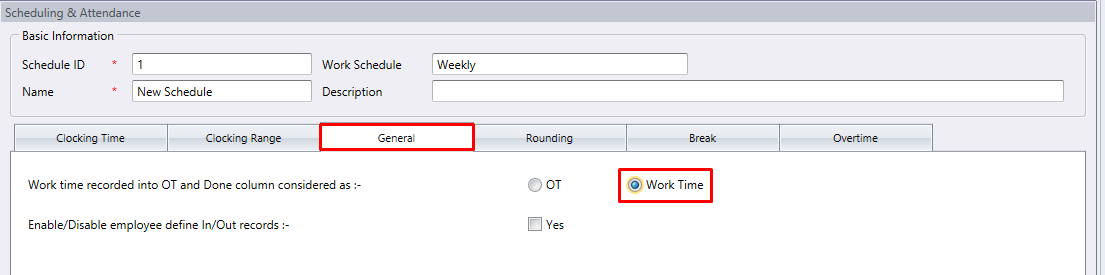Clocking time of user

 Number of clocking 1st 2nd 3rd 4th 5th 6th Cloking time 09:00 12:39 13:15 16:15 19:05 23:40

Result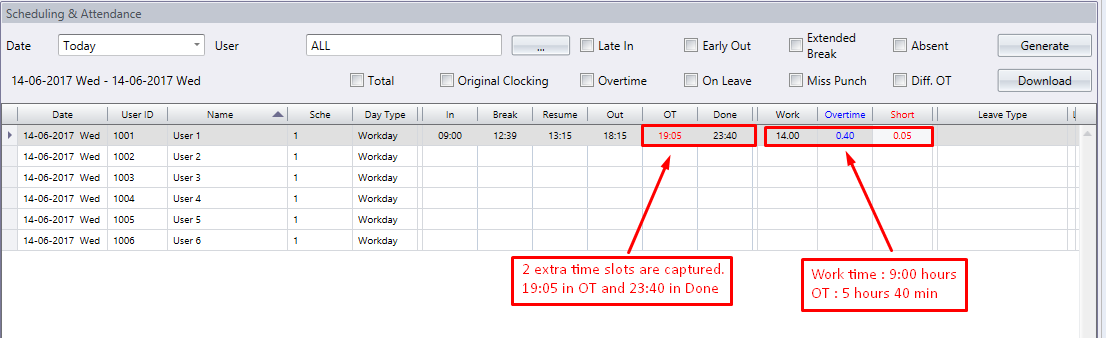The calculation of Ingress/TCMS of work time and OT will change, if the option “Work time recorded into OT and Done column considered as” is set to Work.

Now the OT and Done column will become scheduled work columns as IN   and OUT do. User will have 2 break times in a working day. First break will be from 12:30 to 13:30, and second break will be from 18:00 to 19:00.

Now the Work time is calculated as below,
Work time = Done time – In time

If Actual Done time of user > Preset Done time
Work time = Preset Done time – actual in time
Where work time = 18:00 – 09:00
= 9 hours
If Actual Done time of user < Preset Done time
Work time = Actual Done time – Actual In time

In this example, Done time is 23:40 (> preset Done time), therefore
Work time = Preset Done time – Actual In time
= 23:00 – 14:00
= 14 hours

Since the Done time is more than preset Done time, Ingress/TCMS will calculate the OT of user.
OT = Actual Done time – Preset Done time
OT = 23:40 – 23:40
= 40 min
Ingress/TCMS V3 will also calculate the Short time of user. Short time will only calculate if user comes in later than preset In time and preset OT time. Late from lunch will not consider as short time.  The calculation is as follow,
Total short time = short time (IN) + short time (OT)
Short time (IN) = Actual In time – Preset In time
= 09:00 – 09:00
= 0 min

Short time (OT) = Actual OT time – Preset OT time
= 19:05 – 19:00
= 5min
Therefore total short time = 0 min + 5 min
= 5min